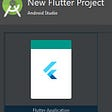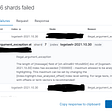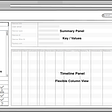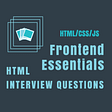# What is Automata?

Automata mean “self-acting”. An automaton (Automata in plural) is an abstract self-propelled computing device that follows a predetermined sequence of operations automatically.

The main concept behind developing the automata theory is to develop methods to describe and analyze the dynamic behaviour of discrete systems.

An automaton with a finite number of states is generally called a Finite Automaton (FA) or Finite State Machine (FSM).

# Finite Automaton:

Finite automata are used to recognize patterns. It takes the string of symbols as input and changes its state accordingly. When the desired symbol is found, then the transition occurs.

At the time of transition, the automata can either move to the next state or stay in the same state.

Finite automata have two states, Accept state or Reject state. When the input string is processed successfully, and the automata reached its final state, then it will accept.

An automaton can be represented by a 5-tuple (Q, ∑, δ, q0, F), where −

• Q is a finite set of states.
• ∑ is a finite set of symbols, called the alphabet of the automaton.
• δ is the transition function.
• q0 is the initial state from where any input is process
• ed (q0 ∈ Q).
• F is a set of final state/states of Q (F ⊆ Q).

# Finite Automata Model:

Finite automata can be represented by input tape and finite control.

Input tape: It is a linear tape having some number of cells. Each input symbol is placed in each cell.

Finite control: The finite control decides the next state on receiving particular input from input tape. The tape reader reads the cells one by one from left to right, and at a time only one input symbol is read.

# What is Büchi automata?

In computer science and automata theory, a deterministic Büchi automaton is a theoretical machine that either accepts or rejects infinite inputs.

Such a machine has a set of states and a transition function, which determines which state the machine should move to from its current state when it reads the next input character.

Some states are accepting states and one state is the start state.

The machine accepts input if and only if it will pass through an accepting state infinitely many times as it reads the input.

## Modelling infinite computations of reactive systems

● An infinite string (⍵ word) α over Σ is a mapping ℕ →Σ and is shown by the infinite sequence a0,a1,a2….

● Set of all ⍵ words over Σ is denoted by Σω = {a1 a2 a3… | ai ϵ Σ}

● A subset L⊆ Σω is called ⍵-language

# Non-deterministic Büchi automaton

In a non-deterministic Büchi automaton, the transition function & is replaced with a transition relation A that returns a set of states, and the single initial state q is replaced by a set of initial states. Generally, the term Büchi automaton without qualifier refers to non-deterministic Büchi automata.

# Operations on Buchi Automata

The standard set operations of non-deterministic Buchi Automata on their languages namely, union, intersection, and complementation, as well as derived operations and decision problems. The main result is that non-deterministic Buchi automata are closed under such operations,

For example, by giving two Buchi automata A0 and A1, we can construct another Buchi automaton A Such that L(A) = L(A0)∩ L(A1). Deterministic Buchi automata, however, are strictly less expressive than non-deterministic ones, since there are

ω-regular languages accepted by a nondeterministic BA for which there does not exist a deterministic BA accepting them; DBAs are also not closed under complementation, i.e there is a DBA whose complement language can only be accepted by a nondeterministic BA.

# Where it is used?

Buchi automata are used in various systems operating systems, air traffic control systems and a factory process control system as these systems have common characteristics to never halt and accept an infinite string of inputs and continue to function.

Blog written by :

Vishal Thoke, Utsav Patel, Sushant Vaidkar and Vedant Valsangkar.

Hope you ❤ it.

--

--

--

## More from Vedant Valsangkar

Love podcasts or audiobooks? Learn on the go with our new app.

## Flutter 101 for React Developers, Mostly… Part I## Monthly Roundup for Holo & Holochain## Essential Skills Require To Become An Android Developer## Elastic search shards failure Error index has exceeded  — maximum allowed to be analyzed…## Keep it Simple and SMART (KiSS) — Engineering Fitness## Effective Algorithms for Dart and Flutter. Hash Set.## 15 General Interview Questions for a Frontend Developer in 2019## All you need to know about Shepard Fairey## Hey! Come watch funny videos with me on ClipClaps!